Triangle:  We just studied polygons. Triangle is a polygon with three sides. So, we could define a triangle as a plane closed figure bounded by three line segments.

A triangle is a polygon with three edges and three vertices. It is one of the most basic shapes in geometry. A triangle with vertices A, B, and C is denoted by ∆ABC.

Kind of Triangles

Classification of triangles based on the length of the sides

 Scalene Triangle A triangle in which all three sides are of different lengths is called Scalene Triangle.   In this type of triangle:$\angle A \neq \angle B \neq \angle C$$\angle A \neq \angle B \neq \angle C$Isosceles Triangle A triangle in which two sides are of the same length is called Isosceles Triangle In this type of triangle:$\angle B = \angle C$$\angle B = \angle C$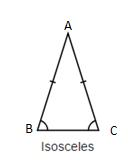Equilateral Triangle A triangle in which all three sides are of the same length is called Equilateral Triangle.   In this type of triangle:$\angle A = \angle B = \angle C$$\angle A = \angle B = \angle C$Some of the diagrams have been adopted from https://en.wikipedia.org/wiki/Triangle

Classification of Triangles based on the angles

 Acute-angled Triangle A triangle in which all the three angles are more than$0^{\circ}$$0^{\circ}$ and less than$90^{\circ}$$90^{\circ}$ is called acute-angled triangle.Right-angles Triangle A triangle in which one of the angles is$90^{\circ}$$90^{\circ}$is called right-angled triangle.Obtuse-angled triangle A triangle in which one of the angles is more than$90^{\circ}$$90^{\circ}$ but less than$180^{\circ}$$180^{\circ}$ is called obtuse-angled triangle.Some of the diagrams have been adopted from https://en.wikipedia.org/wiki/Triangle

Term related to a Triangle

 Median A line segment joining the vertex to the mid-point of the opposite side of a triangle is called median. In this vertex$A$$A$ is meeting at point$D$$D$ (such that$BD=DC$$BD=DC$) midpoint of$BC$$BC$Centroid The point of intersection of three medians is called centroid.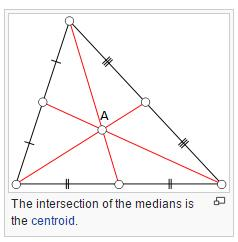Altitude The perpendicular drawn from the vertex to the opposite side. Here$AD$$AD$  is the altitude of the triangle$AD \ and\ BC$$AD \ and\ BC$ is the base.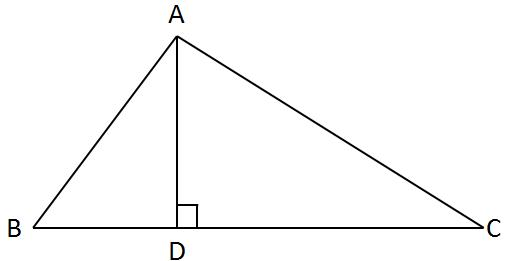Orthocenter The intersection of the three altitudes is called the Orthocenter of the triangle. Here$A$$A$ is the Orthocenter of the triangle.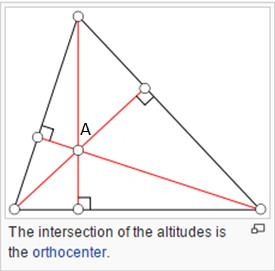Angle Bisector A line segment that bisects and interior angle of a triangle is called angle bisector. Here$AD$$AD$ is bisecting$\angle BAC$$\angle BAC$ into two equal$\angle BAD \ and\ \angle DAC$$\angle BAD \ and\ \angle DAC$Incentre and Incircle The point of intersection of internal angle bisectors is called the Incentre.$I$$I$ is the Incentre of the triangle. Now if you draw a circle with the center$I$$I$  in such a way that it touches all the three sides of the triangle, then that is called Incircle.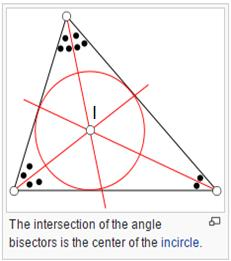Perpendicular Bisector or Right Bisector A line bisecting any side of the triangle and perpendicular to it is called perpendicular bisector of that side of the triangle. Here BC is being bisected by$DE. BD=DC \ and\ ED\perp BC.$$DE. BD=DC \ and\ ED\perp BC.$Circumcenter  and Circumcircle The point of intersection of the perpendicular bisectors of the sides of the triangle is called Circumcenter. Here$O$$O$  is the circumcenter.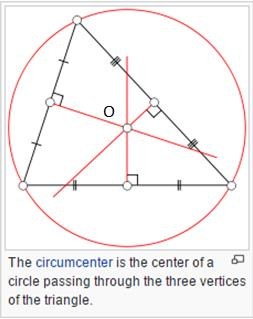Exterior Angle and Interior Opposite Angles of a Triangle$\angle ACD$$\angle ACD$ is the exterior angle and$\angle CBA$$\angle CBA$  and$\angle BAC$$\angle BAC$  are opposite interior angles of this exterior angle.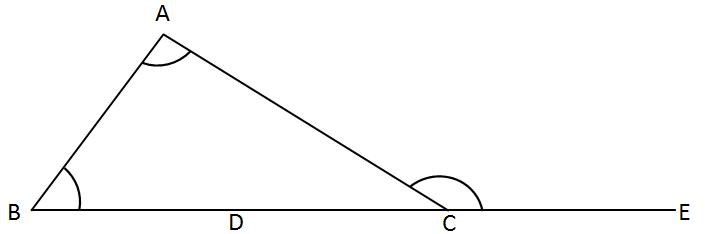Some of the diagrams have been adopted from https://en.wikipedia.org/wiki/Triangle